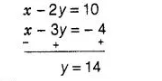# Two years ago, Salim was thrice as old as

Question:

Two years ago, Salim was thrice as old as his daughter and six years later, he will be four year older than twice her age. How old are they now?

Solution:

Let Salim and his daughter’s age be x and y yr respectively.

Now, by first condition

Two years ago, Salim was thrice as old as his daughter.

i.e. $\quad x-2=3(y-2) \Rightarrow x-2=3 y-6$

$\Rightarrow \quad x-3 y=-4$ ....(i)

and by second condition, six years later. Salim will be four years older than twice her age.

$x+6=2(y+6)+4$

$\Rightarrow \quad x+6=2 y+12+4$

$\Rightarrow \quad x-2 y=16-6$

$\Rightarrow \quad x-2 y=10$ ......(ii)

On subtractina Eq. (i) from Eq. (ii), we getPut the value of $y$ in Eq. (ii), we get

$x-2 \times 14=10$

$\Rightarrow \quad x=10+28 \Rightarrow x=38$

Hence, Salim and his daughter’s age are 38 yr and 14 yr, respectively.